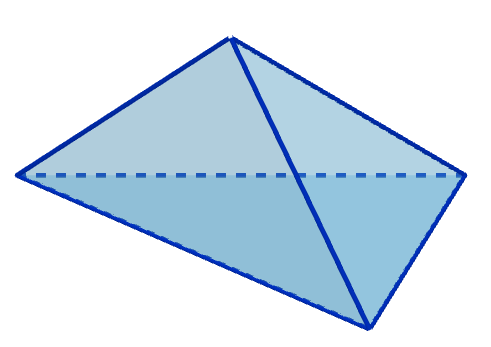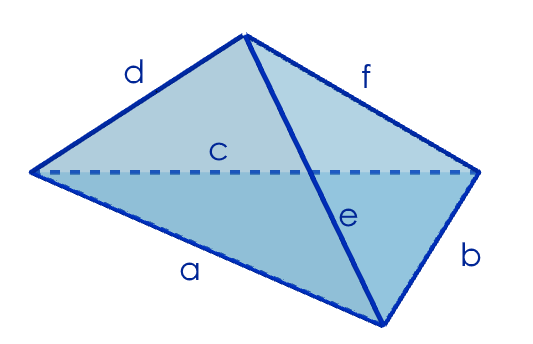#### You may also like### Overturning Fracsum

Solve the system of equations to find the values of x, y and z: xy/(x+y)=1/2, yz/(y+z)=1/3, zx/(z+x)=1/7### Rudolff's Problem

A group of 20 people pay a total of Â£20 to see an exhibition. The admission price is Â£3 for men, Â£2 for women and 50p for children. How many men, women and children are there in the group?### Polycircles

Show that for any triangle it is always possible to construct 3 touching circles with centres at the vertices. Is it possible to construct touching circles centred at the vertices of any polygon?

# Building Tetrahedra

##### Age 14 to 16Challenge Level

Imagine a tetrahedron made up of four triangles which have the same perimeter.
What might it look like?You could start to explore by choosing a triangle with a perimeter of 20, say, and use it as the base of your tetrahedron.

Can you find possibilities for the other three triangles if they all have perimeter 20 too?

Of course, if the triangles are all congruent, the perimeters will definitely be equal!
Can you find an example where the triangles aren't all congruent? Can you prove it?

Click below to see a diagram that might help:Can you write down expressions for the different perimeters?
What can you deduce if you know these perimeters are all equal?

Can you make a tetrahedron out of any set of four congruent triangles?